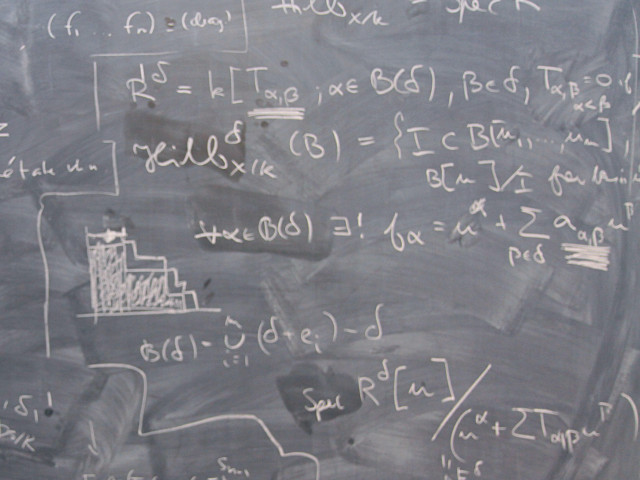# SF1621 Analytical Methods and Linear Algebra II 12.0 creditsBasic course in introductory linear algebra and calculus of several variables with applications.

Course offering missing for current semester as well as for previous and coming semesters
Headings with content from the Course syllabus SF1621 (Autumn 2008–) are denoted with an asterisk ( )

## Content and learning outcomes

### Course contents

After the course, the students should be able to

• Define the fundamental concepts: the vector space Rn, linear dependence and independence, linear transformation, eigenvalue and eigenvector, vector-valued functions, partial derivatives, gradient, directional derivative, differentiability, Jacobian matrix and Jacobian determinant multiple integral, surface integral, line integral, curl, divergence.
• Apply the method of least squares for solving over-determined system of equations.
• Find eigenvalues and corresponding eigenvectors and use them in diagonalization of matrixes and to classify conics and quadric surfaces.
• Transform expressions for derivatives under a change of coordinates and use them to solve some partial differential equations.
• Use the gradient to find directional derivatives and tangent planes to level surfaces.
• Evaluate some multiple integrals, line integrals and surface integrals.
• Find the areas and volumes by using multiple integrals and find the length of a curve using integrals.
• Solve maximum-minimum problems for functions of several variables, also constrained.
• Derive some formulas and theorems.

### Intended learning outcomes

To found a basis for further studies within the engineer education by

• providing practical mathematical skills in using introduced terms
• developing a trust in the own ability to use mathematics
• presenting different methods of proof and illustrating the deductive character of mathematics.
• practicing the ability to communicate with the language and symbols of mathematics
• developing the ability to formulate and analyze relevant problems using mathematical terms
• practicing the ability to follow and carry out logical and mathematical reasoning
• contributing the satisfaction of mastering mathematical terms and methods and in experiencing the beauty and logics of mathematics

After the course, the students should be able to

Fundamental concepts

use the fundamental concepts of linear algebra and calculus of several variables: the vector spaces Rn, basis, linear transformation, eigenvalue, eigenvector, limit of a function of several variables, differentiability, partial derivative, gradient, multiple integral, surface integral, line integral, curl, divergence.

Usage of language

communicate using the language and symbols of mathematics.

Reasoning

perform mathematical reasoning using: implications, equivalences.

Mathematical modeling

set up mathematical models and problems expressed in terms of the fundamental concepts.

Problem solving

use classical solution methods of linear algebra and calculus.

Complementary aims

After the course the students should have

• Enhanced their study technique so that it is well adjusted to the learning of the mathematical scientific and technical subjects.
• Insights on how mathematical tools and thinking can be used in the further education and future professional life.

### Course disposition

No information inserted

## Literature and preparations

### Specific prerequisites

To be able to profit by the course, the student should have the previous knowledge corresponding to SF1622 Calculus in one variable and linear algebra.

### Recommended prerequisites

No information inserted

### Equipment

No information inserted

### Literature

E. Petermann, Linjär geometri och algebra. ISBN 91-44-02119-4.
E. Petermann, Analytiska metoder I, 4:e upplagan. ISBN 91-44-01456-2.
Andersson Lennart m.fl. : Linjär algebra med geometri.

## Examination and completion

If the course is discontinued, students may request to be examined during the following two academic years.

A, B, C, D, E, FX, F

### Examination

• TEN1 - Examination, 12.0 credits, grading scale: A, B, C, D, E, FX, F

Based on recommendation from KTH’s coordinator for disabilities, the examiner will decide how to adapt an examination for students with documented disability.

The examiner may apply another examination format when re-examining individual students.

### Other requirements for final grade

The course aims are written with a direction to the grade 3 and will be examined through continuous examination and a written exam (TEN1; 12 cr). It will be up to the coordinating teacher to decide the forms of the continuous examination.

### Opportunity to complete the requirements via supplementary examination

No information inserted

### Opportunity to raise an approved grade via renewed examination

No information inserted

### Examiner

No information inserted

### Ethical approach

• All members of a group are responsible for the group's work.
• In any assessment, every student shall honestly disclose any help received and sources used.
• In an oral assessment, every student shall be able to present and answer questions about the entire assignment and solution.

## Further information

### Course web

Further information about the course can be found on the Course web at the link below. Information on the Course web will later be moved to this site.

Course web SF1621

SCI/Mathematics

### Main field of study

Mathematics, Technology

First cycle